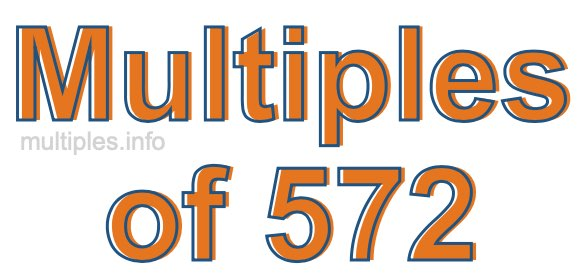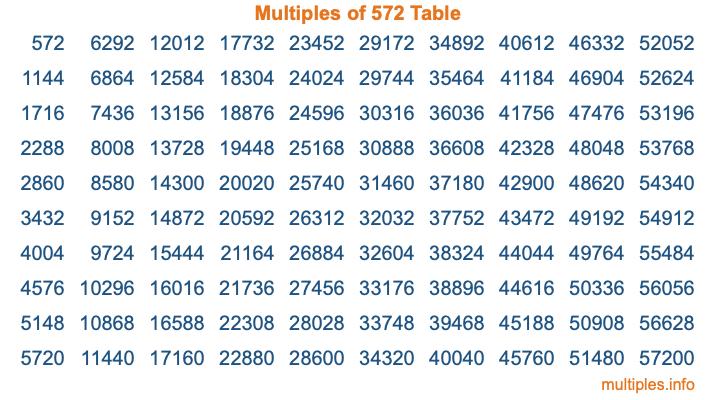Multiples of 572Welcome to the Multiples of 572 page. Here we will first teach you everything you will ever need to know about the multiples of 572, and then give you a study guide summary of everything we taught you to make sure you remember it all. Use this page to look up facts and learn information about the multiples of 572. This page will make you a multiples of five hundred seventy-two expert!

Definition of Multiples of 572
Multiples of 572 are all the numbers that when divided by 572 equal an integer. Each of the multiples of 572 are called a multiple. A multiple of 572 is created by multiplying 572 by an integer.

Therefore, to create a list of multiples of 572, you start with 1 multiplied by 572, then 2 multiplied by 572, then 3 multiplied by 572, and so on for as long as you want. Thus, the list of the first five multiples of 572 is 572, 1144, 1716, 2288, and 2860. To see a larger list of multiples of 572, see the printable image of Multiples of 572 further down on this page. We also have a category where you can choose any nth multiple of 572.

Multiples of 572 Checker
The Multiples of 572 Checker below checks to see if any number of your choice is a multiple of 572. In other words, it checks to see if there is any number (integer) that when multiplied by 572 will equal your number. To do that, we divide your number by 572. If the the quotient is an integer, then your number is a multiple of 572.

Is  a multiple of 572?

Least Common Multiple of 572 and ...
A Least Common Multiple (LCM) is the lowest multiple that two or more numbers have in common. This is also called the smallest common multiple or lowest common multiple and is useful to know when you are adding our subtracting fractions. Enter one or more numbers below (572 is already entered) to find the LCM.

Check out our LCM Calculator if you need more details about the Least Common Multiple or if you need the LCM for different numbers for adding and subtraction fractions.

nth Multiple of 572
As we stated above, 572 is the first multiple of 572, 1144 is the second multiple of 572, 1716 is the third multiple of 572, and so on. Enter a number below to find the nth multiple of 572.

th multiple of 572

Multiples of 572 vs Factors of 572
572 is a multiple of 572 and a factor of 572, but that is where the similarities end. All postive multiples of 572 are 572 or greater than 572. All positive factors of 572 are 572 or less than 572.

Below is the beginning list of multiples of 572 and the factors of 572 so you can compare:

Multiples of 572: 572, 1144, 1716, 2288, 2860, etc.

Factors of 572: 1, 2, 4, 11, 13, 22, 26, 44, 52, 143, 286, 572

As you can see, the multiples of 572 are all the numbers that you can divide by 572 to get a whole number. The factors of 572, on the other hand, are all the whole numbers that you can multiply by another whole number to get 572.

It's also interesting to note that if a number (x) is a factor of 572, then 572 will also be a multiple of that number (x).

Multiples of 572 vs Divisors of 572
The divisors of 572 are all the integers that 572 can be divided by evenly. Below is a list of the divisors of 572.

Divisors of 572: 1, 2, 4, 11, 13, 22, 26, 44, 52, 143, 286, 572

The interesting thing to note here is that if you take any multiple of 572 and divide it by a divisor of 572, you will see that the quotient is an integer.

Multiples of 572 Table
Below is an image of the first 100 multiples of 572 in a table. The table is in chronological order, column by column. The first column has the first ten multiples of 572, the second column has the next ten multiples of 572, and so on.The Multiples of 572 Table is also referred to as the 572 Times Table or Times Table of 572. You are welcome to print out our table for your studies.

Negative Multiples of 572
Although not often discussed or needed in math, it is worth mentioning that you can make a list of negative multiples of 572 by multiplying 572 by -1, then by -2, then by -3, and so on, to get the following list of negative multiples of 572:

-572, -1144, -1716, -2288, -2860, etc.

Multiples of 572 Summary
Below is a summary of important Multiples of 572 facts that we have discussed on this page. To retain the knowledge on this page, we recommend that you read through the summary and explain to yourself or a study partner why they hold true.

There are an infinite number of multiples of 572.

A multiple of 572 divided by 572 will equal a whole number.

572 divided by a factor of 572 equals a divisor of 572.

The nth multiple of 572 is n times 572.

The largest factor of 572 is equal to the first positive multiple of 572.

572 is a multiple of every factor of 572.

572 is a multiple of 572.

A multiple of 572 divided by a divisor of 572 equals an integer.

572 divided by a divisor of 572 equals a factor of 572.

Any integer times 572 will equal a multiple of 572.

Multiples of a Number
Here you can get the multiples of another number, all with the same attention to detail as we did for multiples of 572 on this page.

Multiples of
Multiples of 573
Did you find our page about multiples of five hundred seventy-two educational? Do you want more knowledge? Check out the multiples of the next number on our list!